## SOCNET@LISTS.UFL.EDU

#### View:

 Message:[First|Previous|Next|Last] By Topic:[First|Previous|Next|Last] By Author:[First|Previous|Next|Last] Font: Proportional Font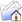LISTSERV Archives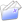SOCNET HomeSOCNET February 2011

Subject:From:Date:Wed, 23 Feb 2011 19:34:26 +0100

Content-Type:text/plain

Parts/Attachments: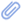text/plain (46 lines)
 ```***** To join INSNA, visit http://www.insna.org ***** Dear Socnet Members Let me address a question to those of you who have been watching developments in GRAPH THEORY. Is the following statement true? 'No nontrivial complete set collection of invariants has yet been found for directed graphs Definitions A (finite) DIRECTED GRAPH is a mathematical object of the form G=(X,R), where X is a finite set (of points) and R is a binary relation in X. Two digraphs G=(X,R) and G=(X,R) are ISOMORPHIC if there exists a 1-1 mapping F of X onto X such that xRy if and only if F(x)RF(y), for any x,y in X. A STRUCTURAL PARAMETER of a digraph is any mapping P which assigns a numerical value to any digraph in such a way that P(G)=P(G) if G and G are isomorphic. A COMPLETE SET OF INVARIANTS is any finite collection P1,,Pk of structural parameters such that, for any two digraphs G and G, the equations P1(G)=P1(G),,Pk(G)=Pk(G) imply that G and G are isomorphic. For any n, let gn denote the number of equivalence classes of the isomorphism relation in the set of digraphs with X={1,,n}. The numbers 1,,gn can be used as code symbols of equivalence classes. A 'trivial' set of invariants is obtained by assigning to any G=(X,R) the number n of points (n=|X| ) and an appropriate symbol from the range from 1 to gn. Hence, by saying that a set of invariants is NONTRIVIAL I mean that the values of P1,,Pk for two n-point digraphs G and G can be computed without prior knowledge of the list of isomorphism classes. Tad Sozanski www.cyf-kr.edu.pl/~ussozans/ _____________________________________________________________________ SOCNET is a service of INSNA, the professional association for social network researchers (http://www.insna.org). To unsubscribe, send an email message to [log in to unmask] containing the line UNSUBSCRIBE SOCNET in the body of the message.```

#### Search Archives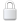Log In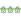Get Password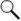Search Archives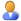Subscribe or Unsubscribe